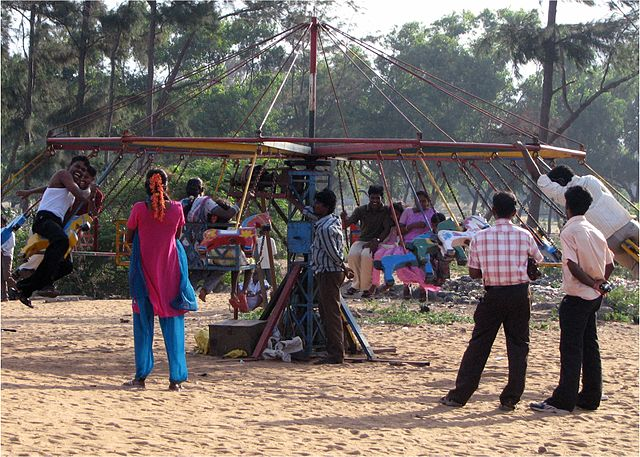Discrete Mathematics

# Geometric Probability: Level 4 Challenges

A number is uniformly chosen from $$[0.15, 0.25]$$. It was rounded to two decimal places and then to one decimal place. The probability that the final value is $$0.2$$ is $$X \%$$. What is $$X?$$

Assumption: Use rounding "half away from zero". That is, if the number is equally far from the two closest numbers, choose the one away from zero. For example, 2.5 is equally far from 2 and 3, so round 2.5 to 3.

Two points are chosen randomly and uniformly along a stick of length $$1.$$ The stick is cut at those points to form $$3$$ pieces. What is the probability that these pieces can form a triangle?7 Sumo Wrestlers are eager to hop onto the merry-go-round. However, if they were to be contained within the same half of the merry-go-round, then their combined weight would cause the merry-go-round to topple.

If all 7 Sumo Wrestlers were to hop onto the merry-go-round at a random point along the circumference of the merry-go-round, what is the probability (to 3 decimal places) that the merry-go-round will topple?

If 3 real numbers are chosen randomly and uniformly from $$[0,1],$$ what is the probability that the square of one of the numbers will be greater than the sum of the squares of the other two numbers?

Three points are chosen uniformly at random from the boundary of a square and a fourth point is chosen uniformly at random from the interior. The probability that the 4th point lies in the triangle formed by the other 3 points can be expressed as $$\frac{a}{b}$$ where $$a$$ and $$b$$ are coprime positive integers. What is the value of $$a + b?$$

×

Problem Loading...

Note Loading...

Set Loading...